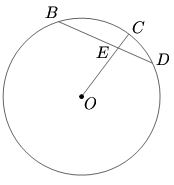$DEB$ is a chord of a circle such that $DE=3$ and $EB=5 .$ Let $O$ be the center of the circle. Join $OE$ and extend $OE$ to cut the circle at $C.$ Given $EC=1,$ find the radius of the circleThe solution for this problem is available for $0.99. You can also purchase a pass for all available solutions for$99.